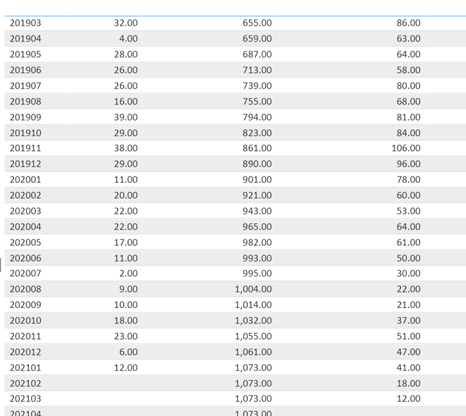cancel
Showing results for
Did you mean:Helper III

## Rolling Sum of a Cumulative Total

Hello again.

Still cannot figure out the DAX to solve my issue:

First column is yearmonth

Second column is monthly sales

Third column is cumulative sales since the launch of the product (e.g., 659 = 655+4), calculated via the following Measure:

Cumulative_Sales = CALCULATE('FACT'[Sales],FILTER(ALLSELECTED('FACT'),'Calendar'[Date]<=max('Calendar'[Date])))

Fourth column is rolling 3 month sum of Second column (e.g. 64 = 28+4+32), calculated via the following Measure

3M_Rolling_Sales =
CALCULATE (sum('FACT'[Sales]),DATESINPERIOD ('Calendar'[Date],LASTDATE ( 'Calendar'[Date] ),-3,MONTH))

Fifth column ... WHAT IS THE MEASURE'S EXPRESSION for the 3M_Rolling_CUMULATIVE_Sales? I cannot generate it
3M_Rolling_CUMULATIVE_Sales ... i.e. for 201905 ... I should have: 687+659+655 = 2,0011 ACCEPTED SOLUTIONSuper User

Sorry, I'm not sure what you mean. I believe the measure creates a cumulative sum of your rolling 3 month sales.

"Fifth column ... WHAT IS THE MEASURE'S EXPRESSION for the 3M_Rolling_CUMULATIVE_Sales?"

Isn't that what you need?In doing so, you are also helping me. Thank you!

Proud to be a Super User!

6 REPLIES 6Super User

Try:

``````3 Month Cumulative =
SUMX (
SUMMARIZE (
FILTER (
ALL ( 'Calendar' ),
'Calendar'[Date] <= MAX ( 'Calendar'[Date] )
),
'Calendar'[YearMonth],
"3MonthRSales", [3M_Rolling_Sales]
),
[3MonthRSales]
)``````

In doing so, you are also helping me. Thank you!

Proud to be a Super User!Helper III

Getting close: This created the cumulative sum of the total column since product launch. How limit that to a 3 month rolling sum ?Super User

Sorry, I'm not sure what you mean. I believe the measure creates a cumulative sum of your rolling 3 month sales.

"Fifth column ... WHAT IS THE MEASURE'S EXPRESSION for the 3M_Rolling_CUMULATIVE_Sales?"

Isn't that what you need?In doing so, you are also helping me. Thank you!

Proud to be a Super User!Helper III

Awesome ! Actually it worked great. Previous issue was myy mistake. Was taking the wrong variable !!Super User

@JMSNYC , Create a measure like this with date table and try

Rolling 3 = CALCULATE(sum(Sales[Sales Amount]),DATESINPERIOD('Date'[Date ],MAX('Date'[Date ]),-3,MONTH))

!! Microsoft Fabric !!
Microsoft Power BI Learning Resources, 2023 !!
Learn Power BI - Full Course with Dec-2022, with Window, Index, Offset, 100+ Topics !!
Did I answer your question? Mark my post as a solution! Appreciate your Kudos !! Proud to be a Super User! !!Helper III

Unfortunately, it does not work. This is the formula I used in Column 4 (with LASTDATE vs. MAX), but still gives me same output 😞